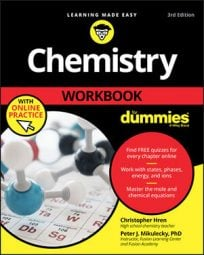##### Chemistry Workbook For Dummies with Online PracticeWhen doing math in chemistry, you need to follow some rules to make sure that your sums, differences, products, and quotients honestly reflect the amount of precision present in the original measurements. That’s where significant figures come in.

You can be honest (and avoid the skeptical jeers of surly chemists) by taking things one calculation at a time, following a few simple rules. One rule applies to addition and subtraction, and another rule applies to multiplication and division.

• Adding or subtracting: Round the sum or difference to the same number of decimal places as the measurement with the fewest decimal places. Rounding like this is honest, because you’re acknowledging that your answer can’t be any more precise than the least-precise measurement that went into it.
• Multiplying or dividing: Round the product or quotient so that it has the same number of significant figures as the least-precise measurement—the measurement with the fewest significant figures.
Notice the difference between the two rules. When you add or subtract, you assign significant figures in the answer based on the number of decimal places in each original measurement. When you multiply or divide, you assign significant figures in the answer based on the smallest number of significant figures from your original set of measurements.

Here’s an example. Express the following sum with the proper number of significant figures:

35.7 miles + 634.38 miles + 0.97 miles = ?

The answer is 671.1 miles. Adding the three values yields a raw sum of 671.05 miles. However, the 35.7 miles measurement extends only to the tenths place. Therefore, you round the answer to the tenths place, from 671.05 to 671.1 miles.

Now let’s try an example with multiplication. Express the following product with the proper number of significant figures:

27 feet * 13.45 feet = ?

Of the two measurements, one has two significant figures (27 feet) and the other has four significant figures (13.45 feet). The answer is therefore limited to two significant figures. You need to round the raw product, 363.15 feet². You could write 360 feet², but doing so may imply that the final 0 is significant and not just a placeholder. For clarity, express the product in scientific notation, as 3.6 * 10² feet².